Next: Probabilistic Encryption Up: A probabilistic test for Previous: A probabilistic test for   Contents

## Analysis of the Rabin test

We will calculate how many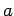are witnesses that 247 is not a prime. Our analysis will make use of the fact that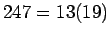and that 2 is a primitive root for both 13 and 19. However, it should be emphasized that this information (which will not be available in general) was not used when we did the test itself.

How manysatisfy? We must haveand. Let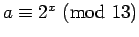. Then we must havedivisible by 12. This gives the possible values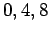for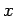, which implies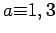, or 9 mod 13. Similarly, if,must be divisible by 18, which leads to, or 11 mod 19. (we actually found 178 above by solving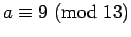and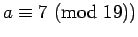The 3 choices mod 13 and mod 19 imply there are 9with.

How manysatisfy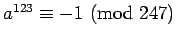? If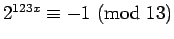, we must have, which leads to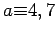, or 17 mod 13. Similarly, we get, or 12 mod 19. Thus we get 9satisfying this condition.

If we choose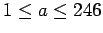at random, the chances of getting anthat is not a witness are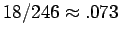. If we do the test 5 times, the chance of incorrectly concluding 247 is a prime is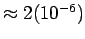.

[We actually did more work than necessary, identifying the exact set of numbers which would lead to a wrong conclusion. If we only want to count how many numbers there are, we could make use of observations such as that, for any, an equation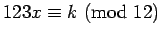will either have 3 solutions or no solutions.]

Theorem 19 (Rabin)   If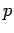is not a prime, at leastof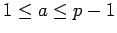are witnesses.

This implies that for any non-prime, the chance of being incorrectly identified after 5 tests is.Next: Probabilistic Encryption Up: A probabilistic test for Previous: A probabilistic test for   Contents
Translated from LaTeX by Scott Sutherland
2002-12-14